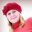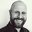Question-and-Answer Resource for the Building Energy Modeling Community
Get started with the Help page

# Scaling in objective definition in JEPLUS+EAI am trying to set up an optimization run with JEPLUS+EA using RVX.

However, I do not understand what the option scaling means in the objective definition. I have read it several time in the JEPLUS help but cannot get a clue. Maybe because I am not a programmer.

Can anyone explain it in simple words or using an example? Thanks a lot.

I attach the code from the example file. Why is scaling true for the first objective but not for the second?

"objectives" : [
{
"identifier" : "t1",
"formula" : "v0/1000/3600",
"caption" : "Electricity [kWh]",
"scaling" : true,
"min" : 0,
"max" : 100000,
"weight" : 1.0
},
{
"identifier" : "t2",
"formula" : "v2",
"caption" : "Construction Cost [$/m2]", "scaling" : false, "min" : 0, "max" : 1000, "weight" : 1.0 } ]  edit retag close merge delete ## Comments Which example file are you looking at? The example file I have from version 1.5 has two objective functions, neither of which are scaled. Ah, I see. I'm not sure if that's meant to be a useable RVX or if it's just there to illustrate the possible values. @Jamie Bull, maybe we should ask @Yi Zhang ? However, I cannot tag Yi Zhang :-( ## 1 Answer Sort by » oldest newest most votedI'll try and give an explanation in simple terms of how the scaling works and what the effect is, following the image in the documentation. Here is the image which illustrates objective scaling.Some observations: 1. Scaling can help with the efficiency of certain algorithms, though I'm not familiar enough with NSGA2 (the default algorithm in jEPlus+EA) to know if that applies here. 2. Scaling sets any values which are less than the min to be equal to 0. This prevents an algorithm which is trying to minimise the objective function from ranking solutions where the value is less than the minimum as better than one which is at the minimum. This avoids runaway optimisation to unfeasible values. 3. Scaling also sets the max value at 1 x n where n is the the weight applied to that variable. The weighting here means that minimising this objective is n times more influential on the result. As to why the "Electricity [kWh]" objective is scaled and the "Construction Cost [$/m2]" objective is not in the example file, that is harder to understand. The effect as far as I can see is that the construction cost could potentially be weighted as 1000 times more important by the evolutionary algorithm. Perhaps @Yi Zhang can weigh in on this?

more

@Jamie Bull. @Waseem: That means in this case that all solutions with Electricity below the min (in this case 0 kWh) are not considered? That makes no sense at all! Because all solutions will consume electricity.

If I want to exclude all solutions with electricity above 100 kWh, I have to put scaling TRUE my min value should be 100?

@Xandrika No, if I'm understanding correctly your max value should be 100, otherwise your solutions won't try and reduce any lower than 100 kWh. Your min can be 0 kWh.

"As to why the 'Electricity [kWh]' objective is scaled and the 'Construction Cost [\$/m2]' objective is not in the example file," there isn't any rationale really, because with NSGA2 scaling has absolutely no effect. :-P

This was a legacy option for single objective algorithms.

Thanks, Yi. So does that mean that the weighting has no effect either?